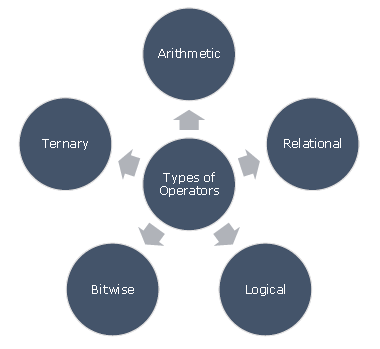# Tcl - Operators

An operator is a symbol that tells the compiler to perform specific mathematical or logical manipulations. Tcl language is rich in built-in operators and provides the following types of operators −

• Arithmetic Operators
• Relational Operators
• Logical Operators
• Bitwise Operators
• Ternary OperatorThis chapter will explain the arithmetic, relational, logical, bitwise, and ternary operators one by one.

## Arithmetic Operators

Following table shows all the arithmetic operators supported by Tcl language. Assume variable ‘A’ holds 10 and variable ‘B’ holds 20, then −

Show Examples

Operator Description Example
+ Adds two operands A + B will give 30
- Subtracts second operand from the first A - B will give -10
* Multiplies both operands A * B will give 200
/ Divides numerator by de-numerator B / A will give 2
% Modulus Operator and remainder of after an integer division B % A will give 0

## Relational Operators

Following table shows all the relational operators supported by Tcl language. Assume variable A holds 10 and variable B holds 20, then −

Show Examples

Operator Description Example
== Checks if the values of two operands are equal or not, if yes then condition becomes true. (A == B) is not true.
!= Checks if the values of two operands are equal or not, if values are not equal then condition becomes true. (A != B) is true.
> Checks if the value of left operand is greater than the value of right operand, if yes then condition becomes true. (A > B) is not true.
< Checks if the value of left operand is less than the value of right operand, if yes then condition becomes true. (A < B) is true.
>= Checks if the value of left operand is greater than or equal to the value of right operand, if yes then condition becomes true. (A >= B) is not true.
<= Checks if the value of left operand is less than or equal to the value of right operand, if yes then condition becomes true. (A <= B) is true.

## Logical Operators

Following table shows all the logical operators supported by Tcl language. Assume variable A holds 1 and variable B holds 0, then −

Show Examples

Operator Description Example
&& Called Logical AND operator. If both the operands are non-zero, then condition becomes true. (A && B) is false.
|| Called Logical OR Operator. If any of the two operands is non-zero, then condition becomes true. (A || B) is true.
! Called Logical NOT Operator. Use to reverses the logical state of its operand. If a condition is true then Logical NOT operator will make false. !(A && B) is true.

## Bitwise Operators

Bitwise operator works on bits and perform bit-by-bit operation. The truth tables for &, |, and ^ are as follows −

p q p & q p | q p ^ q
0 0 0 0 0
0 1 0 1 1
1 1 1 1 0
1 0 0 1 1

Assume if A = 60; and B = 13; now in binary format they will be as follows −

A = 0011 1100

B = 0000 1101

----------------------

A&B = 0000 1100

A|B = 0011 1101

A^B = 0011 0001

The Bitwise operators supported by Tcl language are listed in the following table. Assume variable A holds 60 and variable B holds 13, then −

Show Examples

Operator Description Example
& Binary AND Operator copies a bit to the result if it exists in both operands. (A & B) will give 12, which is 0000 1100
| Binary OR Operator copies a bit if it exists in either operand. (A | B) will give 61, which is 0011 1101
^ Binary XOR Operator copies the bit if it is set in one operand but not both. (A ^ B) will give 49, which is 0011 0001
<< Binary Left Shift Operator. The left operands value is moved left by the number of bits specified by the right operand. A << 2 will give 240, which is 1111 0000
>> Binary Right Shift Operator. The left operands value is moved right by the number of bits specified by the right operand. A >> 2 will give 15, which is 0000 1111

## Ternary Operator

Show Examples

Operator Description Example
? : Ternary If Condition is true? Then value X : Otherwise value Y

## Operators Precedence in Tcl

Operator precedence determines the grouping of terms in an expression. This affects how an expression is evaluated. Certain operators have higher precedence than others; for example, the multiplication operator has higher precedence than the addition operator.

For example : x = 7 + 3 * 2; here, x is assigned 13, not 20 because operator * has higher precedence than +, so it first gets multiplied with 3 * 2 and then adds into 7.

Here, operators with the highest precedence appear at the top of the table, those with the lowest appear at the bottom. Within an expression, higher precedence operators will be evaluated first.

Show Examples

Category Operator Associativity
Unary + - Right to left
Multiplicative * / % Left to right
Additive + - Left to right
Shift << >> Left to right
Relational < <= > >= Left to right
Bitwise AND & Left to right
Bitwise XOR ^ Left to right
Bitwise OR | Left to right
Logical AND && Left to right
Logical OR || Left to right
Ternary ?: Right to left